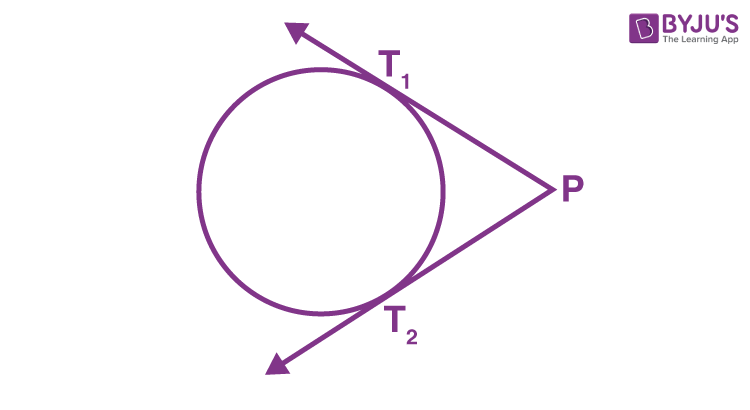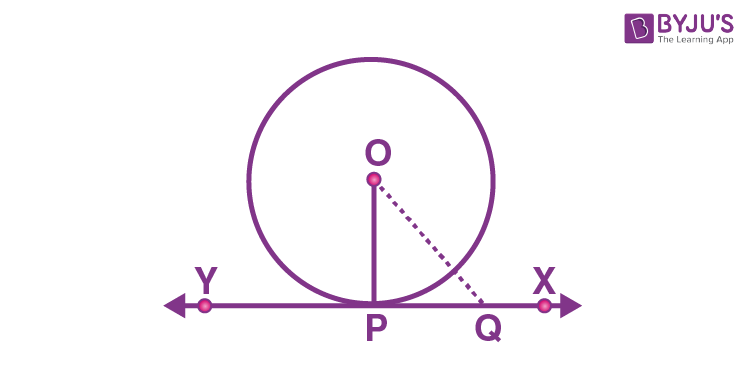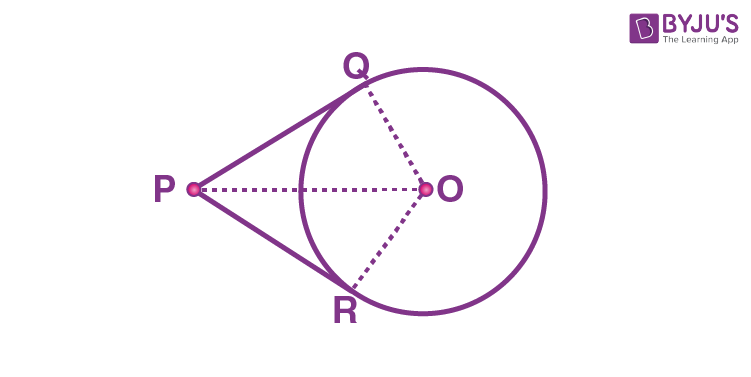# Number of Tangents from a Point on a Circle

In Mathematics, we know that a circle is a closed two-dimensional figure, in which all the points on the surface of a circle are equidistant from the centre point called the radius. The tangent to a circle is a line that intersects the circle at only one point. The point where the circle and the tangent touch is called the point of contact. We can observe three different cases for the number of tangents from a point on a circle. In this article, we will learn the theorem and its proof, and the 3 different cases related to the number of tangents from a point on a circle.

Case 1: No Tangent

There is no tangent to a circle passing through a point that lies inside a circle.Case 2: One Tangent

There is exactly one tangent to a circle passing through a point that lies on a circle.Case 3: Two Tangent

There are exactly two tangents to a circle passing through a point that lies outside the circle.The two tangents observed in this case are PT1 and PT2, and T1 and T2 are the points of contact of the tangents PT1 and PT2, respectively. The length of a tangent from the point P to a circle is the length of the segment of a tangent from the external point P to the point of contact within a circle.

## Tangents From a Point on a Circle Theorem and Proof

The two theorems related to the number of tangents from a point on a circle are explained in detail.

Theorem:

The tangent at any point of a circle is perpendicular to the radius through the point of contact.

Proof:

Consider a circle with centre “O” and XY is the tangent to a circle with the point of contact “P”.

To prove: OP is perpendicular to XY.Take a point Q on the tangent XY other than point P. Now, join the points O and Q.

Note that point Q should lie outside the circle. Because, if the point Q lies inside the circle, XY will become a secant and there is no tangent to a circle.

So, we can say that OQ is greater than OP.

(i.e) OQ > OP

Since the point lies outside of the circle, the above condition is applicable for every point on XY, except the point P.

Thus, OP is the shortest distance of point O to the points on the tangent XY.

Therefore, OP is perpendicular to tangent XY.

Theorem 2:

The lengths of tangents drawn from an external point to a circle are equal.

Proof:

Consider a circle with the centre “O” and P is the point that lies outside the circle. Hence, the two tangents formed are PQ and PR.We need to prove: PQ = PR.

To prove the tangent PQ is equal to PR, join OP, OQ and OR. Hence, ∠OQP and ∠ORP are the right angles.

OP = OP (Common side)

By using the RHS rule, we can say, ∆ OQP ≅ ∆ ORP.

Thus, by using the CPCT rule, the tangent PQ = PR.

Hence, the theorem is proved.

### Examples

Question:

Let TP and TQ are the two tangents drawn to a circle with O from an external point T. Show that ∠PTQ = 2 ∠OPQ.

Solution:

Assume a circle with centre O and the external point is T. Hence, the two tangents formed are TP and TQ to a circle where P and Q are the points of contact as shown in the figure.To prove: ∠PTQ = 2 ∠OPQ.

Let ∠PTQ = θ.

Now, by using the theorem “the lengths of tangents drawn from an external point to a circle are equal”, we can say TP = TQ. So, TPQ is an isosceles triangle.

Therefore,

∠TPQ = ∠TQP = ½ (180°− θ ) = 90° – (½) θ

By using the theorem, “the tangent at any point of a circle is perpendicular to the radius through the point of contact”, we can say ∠OPT = 90°

Therefore,

∠OPQ = ∠OPT – ∠TPQ = 90° – [90° – (½) θ]

∠OPQ = (½)θ

∠OPQ = (½) ∠PTQ, which can also be written as:

∠PTQ = 2 ∠OPQ.

Hence, proved.

Stay tuned with BYJU’S – The Learning App and download the app to explore all Maths-related concepts and learn easily.